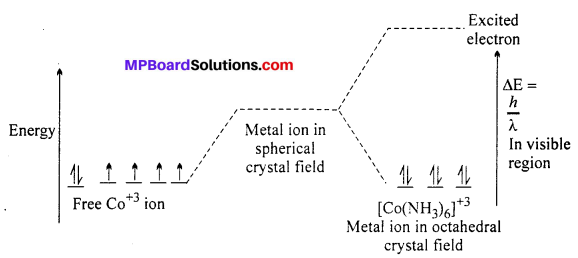# MP Board Class 12th Chemistry Solutions Chapter 9 Coordination Compounds

## MP Board Class 12th Chemistry Solutions Chapter 9 Coordination Compounds

### Coordination Compounds NCERT Intext Exercises

Question 1.
Write the formulas for the following co-ordination compounds :
(i) Tetraamminediaquacobalt (III) chloride
(ii) Potassium tetracyanonickelate (II)
(iii) tris (ethane-1,2-diamine) chromium (III) chloride
(iv) Amminebromidochloridonitrito-N- platinate(II)
(v) Dichlorido 6/s(ethane-1,2-diamine) platinum (IV)nitrate
(vi) Iron(III) hexacyanoferrate(II).
(a) [CO(NH3)4(H2O)2]Cl3
(b) K2[Ni(CN)4]
(c) [Cr(en)3]Cl3
(d) [Pt(NH3)BrCl NO2]
(e) [PtCl2(en)2](NO3)2
(f) Fe4[Fe(CN)6]3.

Question 2.
Write the IUPAC names of the following co-ordination compounds :

1. [CO(NH3)6]Cl3
2. [CO(NH3)5Cl]Cl2
3. K3[Fe(CN)6]
4. K3[Fe(C2O4)3]
5. K2|PdCl4]
6. [Pt(NH3)2Cl(NH2CH3)]Cl.

1. Hexaammine cobalt(III) chloride
2. Pentaamminechloridocobalt(IlI) chloride
3. Potassium hexacyanoferrate(III)
4. Potassium trioxalatoferrate(III)
6. Diamminechlorido(methanamine)platinum(II) chloride.

Question 3.
Indicate the types of isomerism exhibited by the following complexes and draw the structures for these isomers :
(i) K[Cr(H2O)2(C2O4)2]
(ii) [Co(en)3]Cl3
(iii) CO(NH3)5(NO2)](NO3)2]
(iv) [Pt(NH3)(H2O)Cl2].
(i) K[Cr(H2O)2(C2O4)2] shows geometrical isomers :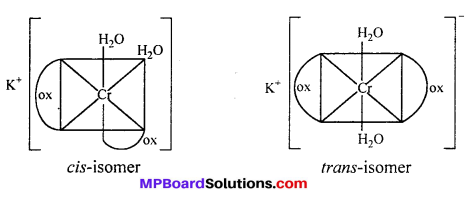cls-isomers exhibits optical isomerism :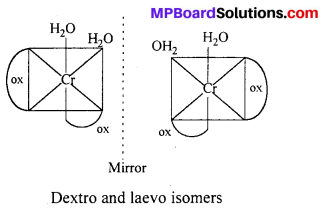(ii) [Co(en)3Cl3] exhibits optical isomerism :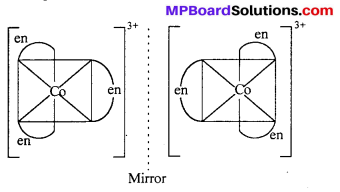(iii) [CO(NH3)5NO2](NO3)2 shows ionisation isomerism [Co(NH3)5NO2](NO3)2 and [Co(NH3)5-NO3](NO2)(NO3). This also shows linkage isomerism.
[CO(NH3)5NO2](NO3)2 and [Co(NH3)5(ONO)] NO3.
(iv) [Pt(NH3)(H2O)Cl2] shows geometrical isomerism.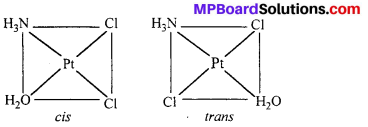Question 4.
Give evidence that [CO(NH3)5Cl]SO4 and [CO(NH3)5SO4]Cl are ionization isomers.
Ionisation isomers when dissolved in water furnish different ions which can be tested.
[CO(NH3)5SO4]Cl + AgNO3 → [CO(NH3)5SO4]NO3 + AgCl ↓
White precipitate indicates that the isomer has Ck ion outside the co-ordination sphere.
[CO(NH3)5Cl]SO4 + BaCl2 → [CO(NH3)5Cl]Cl2 + BaSO4
ppt
White precipitate indicates the isomer has $$\mathrm{SO}_{4}^{2-}$$ ion outside the co-ordination sphere.

Question 5.
Explain on the basis of valence bond theory that [Ni(CN)4]2- ion with square planar structure is diamagnetic and the [NiCl4]2- ion with tetrahedral geometry is paramagnetic.
[Ni(CN)4]2-: The oxidation state of Ni is +2 in the complex. Its electronic cofiguration is [Ar]3d8. It under goes dsp2 hybridization. Because CN being strong ligand causes pairing of electrons.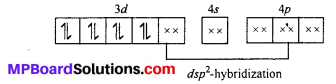[NiCl4]2-: The oxidation state of Ni is +2 in the complex. Its electronic configuration is (Ar)3d8. It undergoes sp3 hybridization. Because Cl being weak field ligand does not causes pairing of electron.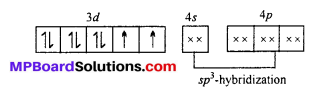Two unpaired electrons are present in the complex ion, hence it is paramagnetic in nature.Question 6.
[NiCl4]2- is paramagnetic while [Ni(CO)4] is diamagnetic though both are tetrahedral. Why ?
[NiCl4]2- has 2 unpaired electrons and is paramagnetic (see Q. No. 5 for details).
Like CN, Co is also strong field ligand, same like Co, it causes pairing of electrons. No unpaired electrons left, hence it is dimagnetic.

Question 7.
[Fe(H2O)6]3+ is strongly paramagnetic whereas [Fe(CN)6]3- is weakly para-magnetic. Explain.
In both the complexes Fe is in +3 oxidation state with the configuration 3d5. CN is a strong ligand. In its presence, 3d-electrons pair up leaving only one unpaired electron. The hybridization is d2sp3 forming inner orbital complex. H2O is a weak ligand. In its presence, 3d-electrons do not pair up. The hybridization is sp3d2 forming an outer orbital complex containing five unpaired electrons. Hence, it is strongly paramagnetic.

Question 8.
Explain [Co(NH3)6]3+ is an inner orbital complex whereas [Ni(NH3)6]2+ is an outer orbital complex.
In [CO(NH3)6]3+, CO is in +3 oxidation state with the configuration 3d6. In the presence of NH3, 3d-electrons pair up leaving two d-orbitals empty. Hence, the hybridization is d2sp3 forming an inner orbital complex.

In [Ni(NH3)6]2+, Ni is in +2 oxidation state with the configuration 3d6. In presence of NH3, the 3d-electrons do not pair up. The hybridization involved is sp3d2 forming outer orbital complex.

Question 9.
Predict the number of unpaired electrons in the square planar [Pt(CN)4]2- ion.
Platinum atom has the ground state electronic configuration 5d4 6s1. In the complex Ft is in +2 oxidation state and has the electronic configuration 5d8. To have square planar geometry, one of the 5d-orbitals is vocated and all the other four orbitals get a pair of electrons. Therefore, it involves dsp2 hybridization and is diamagnetic.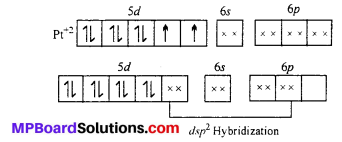Question 10.
The hexaaquomanganese(II) ion contains five unpaired electrons, while the hexacyano ion contains only one unpaired electron. Explain using Crystal Field Theory.
Mn in +2 oxidation state has the electronic configuration 3d5. H2O is a weak ligand. In presence of H2O molecules, the distribution of electrons is $$t^{3}_{2 g} e_{g 2}$$, all the electrons are unpaired.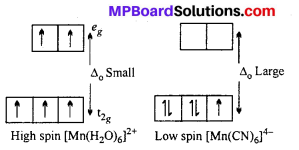CN is a strong ligand. In its presence, the distribution of electrons is $$t_{2 g}^{5}$$ i e one unpaired electron is present.

Question 11.
Calculate the overall complex dissociation equilibrium constant for the Cu(NH3)2+4 ion, given that β4 for this complex is 2.1 × 1013.
Solution:
Overall complex dissociation equilibrium constant is the reciprocal of the over all stability constant.### Coordination Compounds NCERT Textbook Exercises

Question 1.
Explain the bonding in co-ordination compounds in terms of Werner’s pos-tulates.
Werner’s co-ordination theory : Alfred Werner gave his co-ordination theory in 1893. The important postulates of this theory are :
(i) All metals in atomic or ionic form exhibit two types of valencies in co-ordination compounds :
(a) Primary or principal or ionic valency (—–),
(b) Secondary or auxiliary or nonionic valency (—).
The primary valency is ionizable and it is shown by dotted lines. The secondary va-lency is non-ionizable and it is shown by continuous line.
(ii) Primary valency represents oxidation states of metal atom or ion and secondary valency represents co-ordination number of metal ion which is fixed for a particular atom.
(iii) The primary valencies are satisfied by negative ions whereas the secondary valencies may be satisfied either by negative ions (e.g., Cl, Br, CN etc.) or neutral molecules (ag.,H2O).
(iv) Secondary valencies are directed towards fixed position in space.
(v) Eveiy element tends to satisfy both its primary and secondary valencies. For this purpose a negative ion may often act a dual behaviour i.e., it may satisfy primary as well as secondary valency (—–,-).
Example : (i) Luteo cobaltic chloride CoCl3.6NH3 or [Co(NH3)6]Cl3.
(ii) Purpureo cobaltic chloride COC13.5NH3 or [Co(NH3)5Cl]Cl2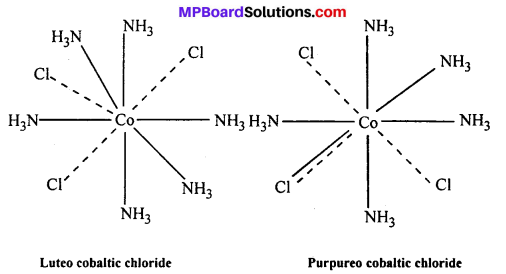Question 2.
FeSO4 solution mixed with (NH4)2SO4 solution in 1:1 molar ratio gives the test of Fe2+ ion but CuSO4 solution mixed with aqueous ammonia in 1:4 molar ratio does not give the test of Cu2+ ion. Explain, why ?
FeSO4 solution mixed with (NH4)2SO4 solution in 1 : 1 molar ratio given double salt, FeSO4(NH4)2.SO4.6H2O (Mohr’s salt). Being double salt, it ionizes in the solution to give Fe2+ ions. Hence, it gives the test of Fe2+ ions. CuSO4 solution mixed with aqueous ammonia in 1 : 4 molar ratio gives a complex compound.
CuSO4 + 4NH3 → [CU(NH3)4]SO4.
The complex ion [Cu(NH3)4]2+ does not ionise to give of Cu2+ ions. Hence, it doesn’t give the test of Cu2+ ions.

Question 3.
Explain with two examples each of the following: co-ordination entity, ligand, co-ordination number, co-ordination polyhedron, homoleptic and heteroieptic.
Co-ordination entity: This entity usually contitutes a central metal atom or ion, to which are attached a fixed number if other atoms or ions or groups by co-ordinate bonds.
e.g. [Ni(CO4)], [CoCl3(NH3)3], etc.
Ligand : It is an ion having at least one lone pair of electrons and capable of forming a co-ordinate bond with central atom/ion in the co-ordination entity.
e.g. Cl, (OH), (CN), etc.
Co-ordination number: The total number of co-ordination bonds with which central atom/ion is linked to ligands in the co-ordination entity is called co-ordination number of central atom/ion.
Co-ordination polyhedron: The spatial arrangement of the ligands which are directly attached to the central atom/ion defines a co-ordination polyhedron about the central atom.
e.g. [CO(NH3)6]3+ is octahedral, [Ni(CO)4] is tetrahedral.
Homoleptic and heteroieptic : Complexes in which a metal is bound to only one kind of donor groups are known as homoleptic.
eg. [CO(NH3)6]3+.
Complex in which a metal is bound to more than one kind of donor groups are called heteroieptic. e.g. [CO(NH3)4Cl2]+

Question 4.
What is meant by unidentate, bidentate and ambidentate ligands ? Give two examples for each.
Unidentate ligand : When a ligand is bound to a metal ion through a single donor atom as with Cl, H2O. The ligand is said to be unidentate.
Didentate ligand : When a ligand is bind through two donor atoms the ligand is said to be didentate.
Example: C2O2-4 (Oxalate)
H2NCH2 – CH2NH2 (ethane 2 diammine)
Ambidentate ligand : Ligand which can ligate through two different atoms is called ambidentate ligand.
Example : -NO2– and SCN.

Question 5.
Specify the oxidation numbers of the metals in the following co-ordination entities:
(i) [Co(H2O)(CN)(en)2]2+
(ii) [CoBr2(en)2]+
(iii) [PtCl4]2-
(iv) K3[Fe(CN)6]
(v) [Cr(NH3)3Cl3].
(a) x + (-1) = +2, x = +3
(b) x + 4(-1) = -2,x = +2
(c) x + 3 (-1) = 0, x = +3
(d) x + 2 (-1) = +1, x = +3
(e) x + 6 (-1) = -3, x = +3.

Question 6.
Using IUPAC norms write the formulas for the following :

1. Tetrahydroxozincate(II)
3. Diamminedichloridoplatinum(II)
4. Potassium tetracyanonickelate(II)
5. Pentaamminenitrito-o-cobalt(III)
6. Hexaamminecobalt(III) sulphate
7. Potassium tri-(oxalato)chromate(III)
8. Hexaammineplatinum(IV)
9. Tetrabromidocuprate(II)
10. Pentaamminenitrito-N-cobalt(III).

1. [Zn(OH]2-
2. K2[PdCl4]
3. [Pt(NH3)2Cl2]
4. K2[Ni(CN)4]
5. [CO(NH3)5(ONO)]2+
6. [CO(NH3)6]2 (SO4)3
7. K3[Cr(C2O4)3]
8. [Pt(NH3)6]4+
9. [Cu(Br)4]2-
10. [CO(NH3)5(NO2)]2+.

Question 7.
Using IUPAC norms write the systematic names of the following :

1. [CO(NH3)6]CI3
2. [Pt(NH3)2Cl(NH2CH3)]Cl
3. [Ti(H2O)6]3+
4. [CO(NH3)4Cl(NO2)]Cl
5. [Mn(H2O)6]2+
6. [NiCl4]2-
7. [Ni(NH3)6]Cl2
8. [Co(en)3]3+
9. [Ni(CO)4].

1. Hexaamminecobalt(III) chloride
2. Diammine chlorido (methylamine) platinum (II) chloride
3. Hexaquatitanium(III) ion
4. Tetraamminechloridonitrito-N-Cobalt(III) chloride
5. Hexaquamanganese(II) ion
6. Tetrachloridonickelate(II) ion
7. Hexaamminenickel(II) chloride
8. tris (ethane-1, 2-diammine) cobalt(III) ion
9. Tetracarbonylnickel (0).

Question 8.
List various types of isomerism possible for co-ordination compounds, giving an example of each.
Isomerism in Co-ordination Compounds : Two or more compounds having the same molecular formula but different arrangement of atoms are-called isomers.
Isomerism in Co-ordination Compounds is given below :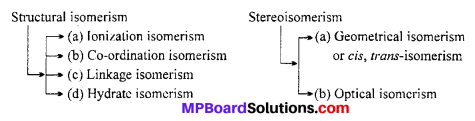1. Structural Isomerism
This type of isomerism arises due to the difference in structures of co-ordination compounds. It is further sub divided into four different types as explained below :

(a) Ionization isomerism : This type of isomerism is shown by such compounds which have same composition but liberate different ions in solution.
Example : [Co(NH3)5Br]SO4 (dark violet). It liberates SO2-4 ions in solution, whereas [Co(NH3)5 SO4] (red) liberates Br- ions in solution. Both of these are ionization isomers.

(b) Co-ordination isomerism : This type of isomerism is shown by such compound which contain both cationic and anionic species and it arises from the inter change of ligands between cationic and anionic entities of different metal ions present in a complex.
For example, [Co(NH3)6][Cr(CN)6] and [Cr(NH3)6][Co(CN)6]
hexaamminecobalt (III) hexacyano chromate (III) and hexaamminechromium (III) hexacyano- cobalt (III).

(C) Linkage isomerism : Linkage isomerism occurs when different atoms of the ligand are attached to the central metal ion. The structure obtained are called linkage isomers. Such type of ligands are called ambidentate ligand.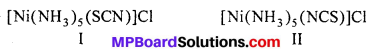In structure I Ni2+ is linked by thiocyanate sulphur and in structure II it is linked by nitrogen atom.
Ionisation Isomerism : This type of isomerism is shown by such compounds which have same composition but liberate different ions in solution.
[Co (NH3)5 Br]SO4 – It liberates SO2-4 ions.
[Co(NH3)5SO4]Br – It liberates Br ions.

(d) Hydrate isomerism : This type of isomerism is shown by such compounds which differ in the number of molecules of water inside the coordination sphere.

Example : The three hydrate isomers of are as follows :

1. [Cr(H2O)6]Cl3 (violet)
2. [Cr(H2O)5Cl]Cl2.H2O (green)
3. [Cr(H2O)4Cl2]Cl.2H2O (darkgreen).

2. Stereoisomerism
Two compounds are called stereoisomers when they contain the same ligand in their co-ordination sphere but differ in their spatial arrangement. Stereoisomerism is further classified as geometrical and optical isomerism.

(a) Geometrical isomerism : When the ligands are situated at different position around the metal it gives rise to geometrical isomerism. The isomer in which similar groups are in adjacent position (making an angle 90° with metal ion) is called c/s-isomer. The iso-mer in which the similar group occupy the opposite position (making an angle of 180° with metal ion) is called trans-isomer.

This type of isomerism is observed in square planar (CN = 4) and octahedral (CN = 6) type of complexes.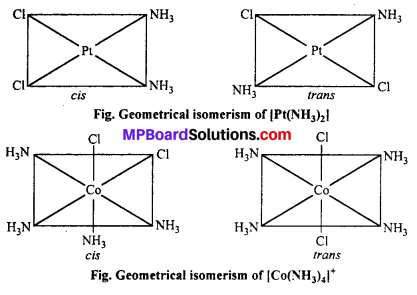(b) Optical Isomerism : Optical isomers are sensitive to plane polarised light. When light passed through the solution of optically active substances these rotates the light-plane in clockwise or anticlockwise direction.

This type of isomerism is exhibited by the substances which are mirror image of each other but cannot be superimposed. Optical isomerism is shown by the substances which have minimum one assymmetric carbon atom.

The compound which rotate plane polarized light in left side are called l-isomers and which rotate in right side called d-isomers. These types of isomerism are generally found in tetrahedral complex [-CN = 4] and octahedral complex [-CN = 6].

(i) Tetrahedral complex : [As (CH3) (C2H5) (S) (C6H4COO)]2+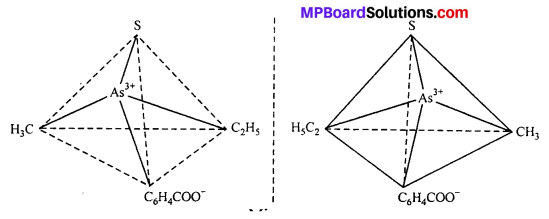(ii) Octahedral complex : [CO(en)2]3+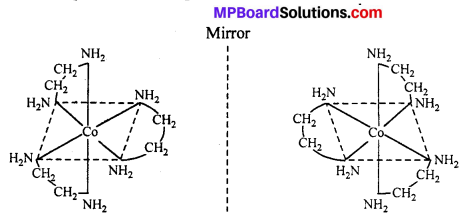Question 9.
How many geometrical isomers are possible in the following co-ordination entities :

1. [Cr(C2O4)3]3-
2. [CO(NH3)3Cl3].

1. Nil
2. Two (fac and mer).

Question 10.
Draw the structures of optical isomers of:
(i) [Cr(C2O4)3]3-
(ii) [PtCl2(en)2]2+
(iii) [Cr(NH3)2Cl2(en)]+.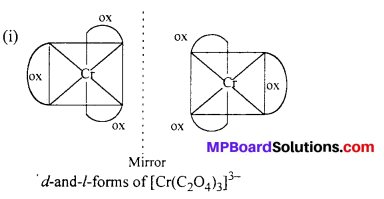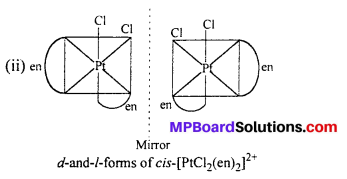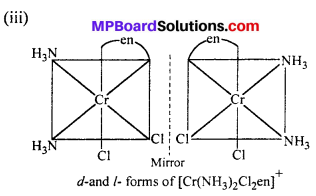Question 11.
Draw all the isomers (geometrical and optical) of:
(i) [CoCl2(en)2]+
(ii) [Co(NH3)Cl(en)2]2+
(iii) [Co(NH3)2Cl2(en)]+.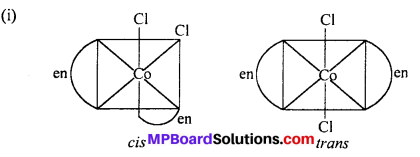cis-isomer will show optical isomer [see question (ii)]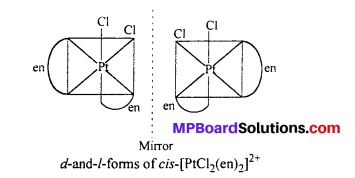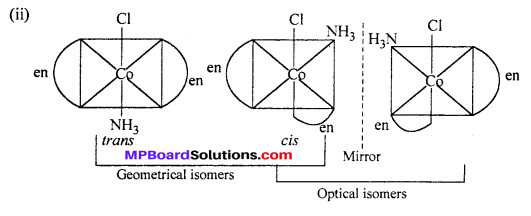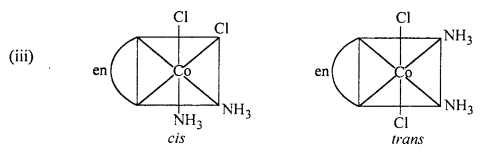cis isomer shows optical isomers

Question 12.
Write all the geometrical isomers of [Pt(NH3)(Br) (Cl)(py)] and how many of these will exhibit optical isomers ?
Three isomers: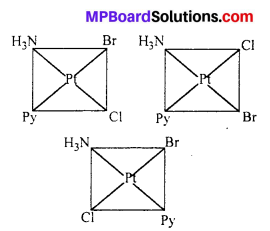These type of isomer do not show optical isomerism. Optical isomerism in square planar complexes occurs only with unsymmetrical chelating ligand.

Question 13.
Aqueous copper sulphate solution (blue in colour) gives:
(i) A green precipitate with aqueous potassium fluoride, and
(ii) A bright green solution with aqueous potassium chloride. Explain these ex-perimental results.
Aqueous copper sulphate exists as [Cu(H2O)4]SO4 which has blue colour due to [CU(H2O)4]2+ ions.

(a) When KF is added, the weak water ligand are replaced by F ligand and forms [CUF4]2+ ions which is green precipitate.
[CU(H2O)4]SO4 + 4F → [CUF4]2- + 4H2O.

(b) When KCl is added, the weak water ligand are replaced by CT ion forming [CuCl4]2- which has bright green colour.
[CU(H2O)4C1] SO4 + 4C1 → [CUC14]2- + 4H2O.

Question 14.
What is the co-ordination entity formed when excess of aqueous KCN is added to an aqueous solution of copper sulphate ? Why is it that no precipitate of copper sulphide is obtained when H2S(g) is passed through this solution ?
When excess of aqueous KCN is added to an aqueous solution of CuSO4, Potassiumtetra-cyanocuprate (II) is formed.
[CU(H2O)4]2+ + 4CN → [CU(CN)4]2- + 4H2O.
When H2S(g) is passed through above solution, no precipitate of copper sulphide is obtained because CN ions are strong ligands so the complex [Cu(CN)4]2- is very stable. As Cu2+ ion are not available so CuS precipitate is not formed.

Question 15.
Discuss the nature of bonding in the following co-ordination entities on the basis of valence bond theory :
(i) [Fe(CN)6]4-
(ii) [FeF6]3-
(iii) [Co(C2O4)3]3-
(iv) [CoF6]3-.
(i) [Fe(CN)6]4- : d2sp3, octahedral, diamagnetic (Refer NCERT Text-book).
(ii) [FeF6]3- : sp3d2, octahedral, paramagnetic (Refer NCERT Text-book) Similar to [CoF6],
(iii) [Co(C2O)4]3- : d2sp3, octahedral, paramagnetic (similar to [Fe(CN)6]4-).
(iv) [CoF6]3- : sp3 d2, octahedral, paramagnetic (Refer NCERT Text-book).

Question 16.
Draw figure to show the splitting of 4-orbitals in an octahedral crystal field.
CFSE can be calculated as :
CFSE = [-0.4x + 0.6y]∆0
Where, ∆0 = CFSE in octahedral complex
x = Number of electrons in t2g orbitals
y = Number of unpaired electrons in eg orbitals.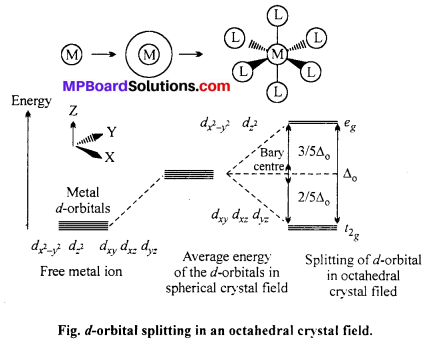Question 17.
What is spectrochemical series ? Explain the difference between a weak field ligand and a strong field ligand.
Spectrochemical series : The arrangement of ligands in order of their increasing field strengths i.e. increasing crystal field splitting energy (CFSE) values is called spectrochemical series, which is as follows :
I < Br < SCN < Cl < S2- < F < OH < C2O2-4 < H2O < NCS < edta-4 < NH3 < en < CN < CO.
Difference between weak field ligand and a strong field ligand : The ligand with small value of CFSE (∆0) are called weak field ligands whereas those with large value of CFSE are called strong field ligands.

Question 18.
What is crystal field splitting energy (CFSE) ? How does the magnitude of ∆0 decide the actual configuration of d-orbitals in a co-ordination entity ?
When ligands approach a transition metal ion, the 4-orbital split into two sets, with lower energy and higher energy. The difference of energy between two sets of orbitals is called crystal field splitting energy (CFSE) i.e. ∆0 for octahedral field.
For example : d4 system has following configuration depending upon ∆0.
(i) If ∆0 < P (pairing energy), the 4th e entres one of the eg orbital giving configuration. $$t_{2 \mathrm{g}}^{4} e_{g 0}$$
(ii) If ∆0 > P, the 4th e paired up in one of the eg orbital giving $$t_{2 \mathrm{g}}^{3} e_{g 1}$$ configuration.

Question 19.
[Cr(NH3)6]3+ is paramagnetic while |Ni(CN)4]2- is diamagnetic. Explain, why ?
[Cr(NH3)6]3+ ion is paramagnetic whereas [Ni(CN4)]2+ is diamagnetic.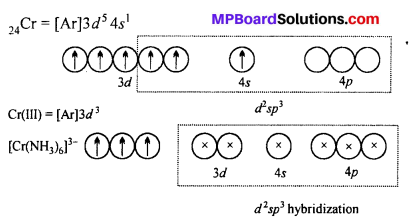Six NH3 ligands donate electrons and form d2 sp3 hybrid orbitals. Since there are three unpaired electrons in 3d subshell. Thus, [Cr(NH3)6]3+ ion is paramagnetic.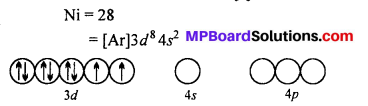CN ion is a strong ligand.
Therefore, it forces the electrons to pair up in 3d subshell and vacant them which participate in hybridization. One 4.9, two 4p and one 4d subshell form dsp2 hybrid orbital.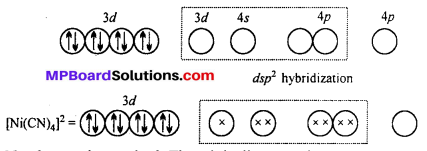Question 20.
A solution of [Ni(H2O)6]2+ is green but a solution of [Ni(CN)4]2- is colourless. Explain.
H2O is a weak ligand [Ni(H2O)6]2- is a outer orbital complex. The complex has two unpaired electron. The d-d transition is possible. It absorbs red light and complemen-tary green light is emitted.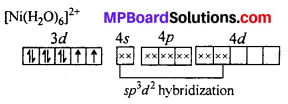CN is a strong ligand. The unpaired electrons are paired up. The central atoms undergoes dsp2 hybridization. Square planar complex is formed. No unpaired electrons are present i. e. d-d transition is not possible, hence the complex is colourless.

Question 21.
[Fe(CN)6]4- and [Fe(H2O)6]2+ are of different colours in dilute solutions, why?
In both the complexes, Fe is in +2 state with the configuration 3d6 i.e., it has four unpaired electrons. As the ligand H2O and CN possess different crystal field splitting energy (∆0), they absorb different components of the visible light (VIBGYOR) for transition. Hence, the transmitted colours are different.

Question 22.
Discuss the nature of bonding in metal carbonyls.
The metal-carbon bonds in metal carbonyls have both s nad p characters. M-C σ-bond is formed by the donation of lone pair of electrons on the carbonyl carbon into a vacant orbital of the metal. M-C π-bond is formed by the donation of a pair of electrons from the filled metal d orbital into the vacant anti-bonding π* orbital of carbon monoxide. This is also known as back bonding of the carbonyl group. The metal to ligand bonding creates a synergic effect which strengthens the bond between CO and the metal. This synergic effect strengthens the bond between CO and the metal.

Question 23.
Give the oxidation state, rf-orbital occupation and co-ordination number of the central metal ion in the following complexes :
(i) K3[CO(C2O4)3]
(ii) cis-[Cr(en)2Cl2]Cl
(iii) (NH4)2[CoF4]
(iv) [Mn(H2O)6]SO4.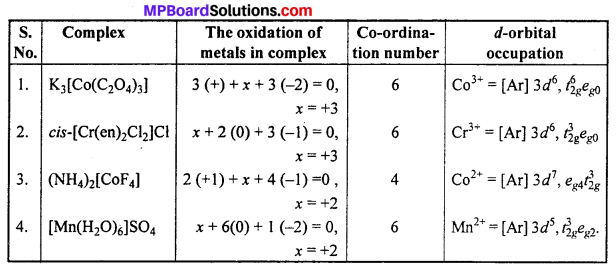Question 24.
Write down the IUPAC name for each of the following complexes and indicate the oxidation state, electronic configuration and co-ordination number. Also give stereochemistry and magnetic moment of the complex :

1. K[Cr(H2O)2(C2O4)2].3H2O
2. [Co(NH3)5Cl]Cl2
3. CrCl3(py)3
4. Cs[FeCl4]
5. K4[Mn(CN)6].

2. Pentaamminechloridocobalt(III) chloride
3. Trichloridotripyridinechromium (III)
4. Caesium tetrachloroferrate (III)
5. Potassium hexacyanomanganate(II).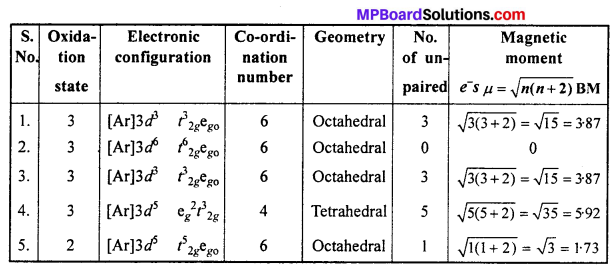Question 25.
What is meant by stability of a co-ordination compound in solution ? State the factors which govern stability of complexes.
The stability of a complex in a solution refers to the degree of association between the two species involved in a state of equilibrium. Stability can be expresed quantitatively in terms of stability constant or formation constant.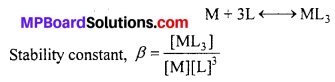For this reaction, the greater the value of the stability’ constant, the greater is the proportion of ML3 in the solution. Stability can be of two types:

(a) Thermodynamic stability : The extent to which the complex will be formed or will be transformed into another species at the point of equilibrium is determined by thermodynamic stability.

(b) Kinetic stability: This helps in determining the speed with which the transformation will occur to attain the state of equilibrium.
Factors affect complex compounds :
(1) Nature of metal ion : The term nature means change density on the central metal ion, greater the charge density more will be the stability of complex.

(2) Size of metal ion :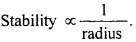(3) Nature of ligand (a) : If the ligand is small in size and its charge is more, the complex will be more stable.

(b) Greater the basicity of ligand, greater the stability of complex.

(4) Chelate ligand : Complex formed by chelate ligand is ten times more stable than ordinary ligand.

(5) Nature of solvent : If a metal and ligand are made to react in a solvent of low dielectric constant and low dipole moment, the stability of the complex increases.

Question 26.
What is meant by the chelate effect ? Give an example.
When a ligand attaches to the metal ion in a manner that forms a ring, then the metal-ligand association is found to be more stable. In other words, we can say that com-plexes containing chelate rings are more stable than complexes without rings. This is known as the chelate effect for example :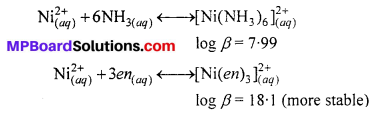Question 27.
Discuss briefly giving an example in each case the role of co-ordination compounds in:
1. Biological system
2. Medicinal chemistry
3. Analytical chemistry
4. Extraction/metallurgy of metals.
1. Biological system : Zinc and iron deficiency of plants is very common. Plants require iron and zinc for healthy growth. When applied to the soil in the form of their saluble salts, these increase metals get precipitated in the from of their hydroxides at pH 7-8 the soil.

To prevent it and their rapid and absorption by the plants, their soluble complexes like iron-EDTA and zinc-EDTA are used.

2. Medicinal chemistry : Many co-ordination complex are used in medicines:
(i) Vitamin B12 which is used to prevent anaemia is a complex compound of cobalt (I), an unusual oxidation atate of cobalt.
(ii) The platinum complex cis-[Pt(NH3)2Cl2] known as cis platin is used in the treatment of cancer. It also inhibits the growth of fiimours. This is known as Chemotherapy.
(iii) The excess of copper and iron are removed by the chelating ligands D-penicil- lamine and desferrioxime-B via the formation of co-ordination compounds.
(iv) The chelate complex of calcium and EDTA is used in the treatment of lead poi¬soning. Inside the body calcium in the complex is replaced by lead. The more soluble lead- EDTA complex is eliminated in urine.

3. Analytical chemistry : Co-ordination compounds find their applications in both qualitative and quantitative methods of analysis.
(a) In qualitative analysis : The formation of complex substance by using suitable reagents is very effectively used in seperation and detection of cations in qualitative analysis. For example :

(i) The seperation of Ag+ from $$\mathrm{Hg}_{2}^{+2}$$ in the first group of analysis is based on the fact that, while silver chloride is soluble in aqueous ammonia and Hg2Cl2 forms back. Insoluble complex which can be seperates by filtration.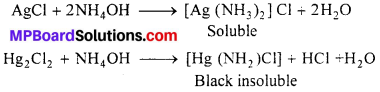(ii) Similarly, seperation of Cd+2 from Cu+2 could also be achieved by treating the solution of Cu+2 and Cd+2 with exess of CN reaction takes place.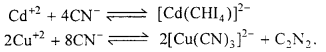Now, when H2S is passed in the solution, ionic product does not exceed the Ksp of Cl2S due to formation of a highly stable complex of copper and hence only CdS gets precipitated.

(b) In quantitive analysis : (i) Gravimetric estimation of Ni+2 is carried out by precipitating Ni+2 as red nickel-dimethyl oxime complex in thr presence of ammonia.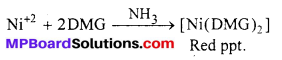(ii) EDTA is a versatile complexing agent. It is used in the complexometric determina¬tion of several metal ions such as Ca+2, Mg+2, Zn+2, Mn+2, Fe+2, Co+2, Ni+2 etc.

4. Extraction/metallurgy of metals : The extraction of silver and gold is done by the formation of cyanide complexes. The crushed ore is treated with an aqueous cyanide solution in the presence of air to dissolve gold by forming the soluble complex ion [Au(CN)2].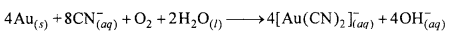The complex is separated and then treated with an electropositive metal, such as zinc to recover gold.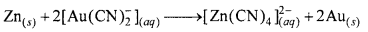The extraction of nickel by means of Mond’s process is based on the preparation of volatile nickel carbonyl. Carbon monoxide gas is passed over impure nickel metal at about 70 °C to form the volatile nickel carbonyl. Distillation of this at 200°C yields nickel.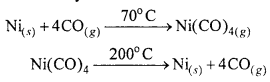Question 28.
How many ions are produced from the complex [Co(NH3)6CI2] in solution :
(i) 6
(ii) 4
(iii) 3
(iv) 2.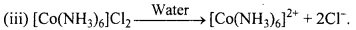Question 29.
Amongst the following ions which one has the highest magnetic moment value :
(i) [Cr(H2O)6]3+
(ii) [Fe(H2O)6]2+
(iii) [Zn(H2O)6]2+.
(ii) [Fe(H2O)6]2+ has the highest magnetic moment because Fe2+ ion has maxi-mum Cl unpaired electrons.

Question 30.
The oxidation number of cobalt in K[Co(CO)4] is :
(i) +1
(ii) +3
(iii) -1
(iv) -3.
(c) K[Co(CO)4]
1 + x + 0 = 0
x = -1.

Question 31.
Amongst the following, the most stable complex is :
(i) [Fe(H2O)6]3+
(ii) [Fe(NH3)6]3+
(iii) [Fe(C2O4)3]3-
(iv) [FeCl6]3-.
(iii) [Fe(C2O4)3]3- is most stable because it is chelate ligand complex.

Question 32.
What will be the correct order for the wavelengths of absorption in the visible region for the following :
[Ni(NO2)6]4-, [Ni(NH3)6]2+, [Ni(H2O)6]2+.
In all the three complexes, the central metal ion is same hence, the increasing field strengths of the ligand from the spectrochemical series are in the order :
H2O < NH3 < NO2
Thus, the energies absorbed for excitation will be in the order :
[Ni(H2O)6]2+ < [Ni(NH3)6]2+ < [Ni(NO2)6]4-.
As E = $$\frac{h c}{\lambda}$$ , the wavelength absorbed will be in opposite order.### Coordination Compounds Other important Questions and Answers

Coordination Compounds Objective Type Questions

Question 1.

Question 1.
The correct structural formula of Zeise’s salt is :
(a) K+ [PtCl3(C2H4)]
(b) K+ [PtCl2(n2 – C2H4]Cl
(c) K2 [PtCl3(n – C2H4)]
(d) K+ [PtCl3(n2 – C2H4)]
(d) K+ [PtCl3(n2 – C2H4)]

Question 2.
Which of the following is not an olefinic organometallic :
(a) C4H4Fe(CO)3
(b) (C2H4PtCl3)2
(c) Be(CH2)2
(d) K[C2H4PtCl3].3H2O.
(c) Be(CH2)2

Question 3.
IUPAC name of K3[Al(C2O4)3] is :
(a) Potassium alumino oxalate
(b) Potassium trioxalato aluminate (III)
(c) Potassium aluminium (III) oxalate
(d) Potassium trioxalato aluminate (IV).
(b) Potassium trioxalato aluminate (III)

Question 4.
AgCl is soluble in aqueous ammonia due to formation of:
(a) [Ag(NH3)2+
(b) [Ag(NH4)2]+
(c) [Ag(NH3 )4 ]+
(d) [Ag(NH3)2]+.
(d) [Ag(NH3)2]+.

Question 5.
Which of the following gives a white precipitate in aqueous solution with silver nitrate :
(a) [Cr(NH3)5Cl](NO2)2
(b) [Pt(NH3)2Cl2]
(c) [Pt(CN)2Cl2]
(d) [Pt(NH3)2]Cl2.
(d) [Pt(NH3)2]Cl2.

Question 6.
Correct nomenclature of Fe4[Fe(CN)6]3 is :
(a) Ferroso ferric cyanide
(b) Ferric ferrous hexacyanate
(c) Iron (III) hexacyanoferrate (III)
(d) Hexa cyano ferrate (III-II).
(c) Iron (III) hexacyanoferrate (III)

Question 7.
Number of geometrical isomers of [Pt(NH3)2Cl2] will be :
(a) Two
(b) One
(c) Three
(d) Four.
(a) Two

Question 8.
In co-ordination compounds, coordination number of metal is :
(a) Equal to primary valency
(b) Sum of primary and secondary valency
(c) Equal to secondary valency
(d) None of these.
(c) Equal to secondary valency

Question 9.
In which of the following complex, oxidation state of metal is zero :
(a) [Pt(NH3)2Cl2]
(b) [Cr(CO)6]
(c) [Cr(NH3)3Cl3]
(d) [Cr(CN)2Cl2].
(b) [Cr(CO)6]

Question 10.
Which of the following is not an organometallic compound :
(a) Ethyl magnesium bromide
(c) Sodium ethoxide
(d) Tetra methyl aluminium.
(c) Sodium ethoxide

Question 11.
Co-ordination number of Fe in [Fe(CN)6]4-, (Fe(CN)6]3- and [Fe(Cl)4] is :
(a) 2, 2, 3
(b) 6, 6, 4
(c) 6, 3, 3
(d) 6, 4, 6.
(b) 6, 6, 4

Question 12.
Example of dsp2 hybridization is :
(a) [Fe(CN)6]3-
(b) [Ni(CN)4]2-
(c) [Zn(NH3)4]2+
(d) [FeF6]3-
(b) [Ni(CN)4]2-

Question 13.
Which of the following complex is used as an anti-cancer agent:
(a) trans[Co(NH3)3Cl3]
(b) cis[Pt(NH3)2Cl2]
(c) cis-K2[PtCl2Br2]
(d) Na2CO3.
(b) cis[Pt(NH3)2Cl2]

Question 14.
Correct order of hybridization of central atoms in NH3.[PtCl4]2- PCl5 and BCl3 is:
(a) dsp2, dsp3, sp2 and sp3
(b) sp3, sp3, sp2 and sp2
(c) dsp2, sp2, sp3 and dsp3
(d) dsp2, sp3, sp2 and dsp3
(b) sp3, sp3, sp2 and sp2

Question 15.
Oxidation state of Fe in Fe(CO)5 complex is :
(a) -1
(b) +2
(c) +4
(d) Zero.
(d) Zero.

Question 16.
Grignard reagent is :
(a) Organometallic compound
(b) Complex compound
(c) Double salt
(d) Neutral compound.
(a) Organometallic compound

Question 17.
Structure of complex salts was proposed by :
(a) Berzelius
(b) Werner
(c) Raoult
(b) Werner

Question 18.
Mohrs salt is :
(a) Double salt
(b) Complex salt
(c) Neutral salt
(d) Reagent.
(a) Double salt

Question 19.
The formula of sodium nitro prusside is :
(a) Na4 [Fe (CN)5 NO5]
(b) Na2 [Fe (CN)5 NO]
(c) NaFe [Fe (CN)6]
(d) Na2 [Fe (CN)6 NO2].
(b) Na2 [Fe (CN)5 NO]

Question 20.
Which of the following compound is different:
(a) Potassium ferrocyanide
(b) Ferrous ammonium sulphate
(c) Potassium ferricyanide
(d) Tetrammine copper (II) sulphate.
(b) Ferrous ammonium sulphate

Question 2.
Fill in the blanks :

1. cis [Pt(NH3)2Cl2] complex is used as an …………………… agent.
2. Haemoglobin is a …………………… compound of iron.
3. Geometrical isomerism is found in both …………………… and …………………… complexes.
4. Oxidation state of Ni in Ni(CO)4 is ……………………
5. Diethyl zinc is a …………………… compound.
6. The correct I.U.P.A.C. name of K4[Fe(CN)6] is ……………………
7. Oxidation state of Co in [Co (E.D.T.A)] is ……………………
8. The formula of dibromo chlorotriaquo chromium (III) is ……………………
9. [COF6]-3 is a spin …………………… complex.
10. The formula of antiknock organo-metallic substance is ……………………
11. E. D. T. A is …………………… ligand.

1. Anti-cancer
2. Complex
3. Tetrahedral, Octahedral
4. Zero
5. Organo-metallic compound
6. Pottassium hexacyano ferrate (II)
7. +3
8. [Cr(H2O)3Cl Br2]
9. High

Question 3.
Match the following :1. (h)
2. (g)
3. (f)
4. (e)
5. (b)
6. (d)
7. (a)
8. (c)
9. (i).

Question 4.

1. Which type of isomerism is found in [Co(NH3)5Br]SO4 and [Co(NH3)5SO4]Br ?
2. Name the organo-metallic compound which is used as an antiknock compound in petrol.
3. Complexes of E.D.T.A. formed with calcium is used to remove the poisoning caused due to which metal ?
4. How is the structure of dibenzene ?
5. What type of hybridization is found in [Ni(CO)4] ?
6. Which type of isomerism is represented by [Cr(H2O)5SCN]2+ and [Cr(H2O)5NCS]2+ ?

1. Ionization isomerism
4. Sandwich
5. sp3Coordination Compounds Short Answer Type Questions

Question 1.
Explain double salt and complex salt. Give one-one example of each.
Double salt: Double salts are additive compounds which are stable in the crys-tal lattice but when dissolved in water break into different compounds.
Example : Ferrous ammonium sulphate is a double salt which ionise in water as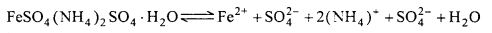Complex salt : The compounds in which ligand with lone-pair electron are linked with any metal atom or metal ion by coordinate bonds, are called coordination compounds. In these compounds metal ion and ligand in combined state act as complex ion and thus these compounds are also known as complex compounds.
Example : K4[Fe(CN)6].

Question 2.
Explain Ambidentate ligand.
Ligands which contain more than one atom to donate electron pair to central metal atom but it donates through one atom at a time are known as ambidentate ligand.
Example : NO2 ion ligand can co-ordinate to central metal atom either through N or by O.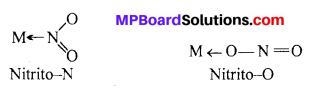Question 3.
What do you understand by ligand ? Explain giving example.
Ligand : Any atom, ion or molecule which can donate electron pair to central ion and forms co-ordinate bond are called ligand. Example : In K4[Fe(CN)6], CN is ligand.

In ligand the specific atom which donates the electron pair is known as donor atom. On the basis of number of donor atoms in a ligand, they are classified as monodentate, bidentate, tridentate, polydentate ligands. Some such ligands are given below :Question 4.
What is complex ion ?
Complex Ion : A complex ion is an electrically charged redical or a species, carrying either positive or negative charge, in which the central metal ion is surrounded through co-ordinate bond by a suitable number of ligands (neutral molecules or negative ions).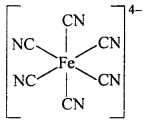Example : Complex ferrocyanide ion[Fe(CN)6]4- is formed by the union of six CN ions with one Fe2+ ion. While writing the formula of a complex ion, the co-ordinating groups are written inside the bracket ( ), and the whole of the complex ion in a square bracket [ ]. The net charges is written on right hand top comer of the square bracket.

The square bracket is known as co-ordination sphere.

Question 5.
Give example of bidentate and hexadentate ligand.
Bidentate ligand :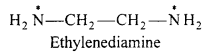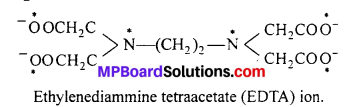Question 6.
What is co-ordination number ? Give any two examples.
Co-ordination number: Number of ligands which are directly linked with central metal or metal ion by co-ordinate bonds, are co-ordination number.
Example : (i) [CO(NH3)6]Cl3 co-ordination number of CO3+ ion is 6.
(ii) In [Ag(CN)2] co-ordination number of Ag is 2.

Question 7.
What are organometallic compounds ? Write its two applications.
Those compounds in which the carbon atom of organic groups are directly bonded to metal atoms are called organometallic compound. The compound of element such as boron, phosphorus, silicon, germanium and antimony with organic groups are also included in the organometallics.

Applications of organometallic compounds :

1. Tetra ethyl lead (C2H5)4 Pb is used as on antiknock compound.
2. Ziegler-Natta catalysis is used in the polymerization of ethylene or other alkenes.
3. Ethyl mercuric chloride (C2H5HgCl) is used in agriculture as an insecticide.
4. Willkinson catalyst is used in the hydrogenation of some alkenes.

Question 8.
Explain geometrical isomerism with an example.
Geometrical isomerism : When the ligands are situated at different position around the metal it gives rise to geometrical isomerism. The isomer in which similar groups are in adjacent position (making an angle 90° with metal ion) is called c/s-isomer. The iso-mer in which the similar group occupy the opposite position (making an angle of 180° with metal ion) is called trans-isomer.

This type of isomerism is observed in square planar (CN = 4) and octahedral (CN = 6) type of complexes.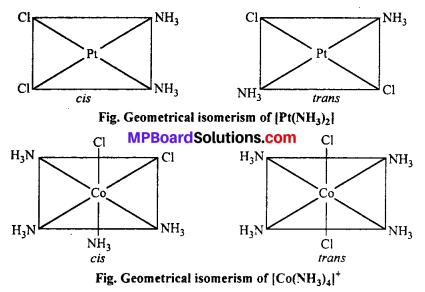Question 9.
Explain the principle of Werner with the example of K4[Fe(CN)6] complex.
The various terms used for a co-ordination compound are shown in the following example: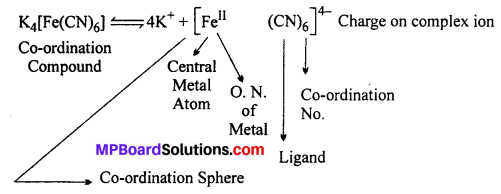(i) When it is dissolved in water it does not break into its constitutent ions K+, Fe2+, CN but it forms K+ and complex ion [Fe(CN)6]4-.
(ii) IUPAC name of the compound is Potassium hexacyanoferrate (II).
(iii) Ionisation of K4Fe(CN)6 in aqueous solution.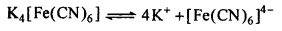According to Werner’s theory : Central metal atom in the compound is Fe whose oxidation number (Primary valency) is 4 and co-ordination number (Secondary valency) is 6.

Question 10.
Explain structure of [Ni(CN)4]2- on the basis of valence bond theory.
In [Ni(CN)4]2- ion, Nickel is present as Ni2+ ion and its valency shell configuration is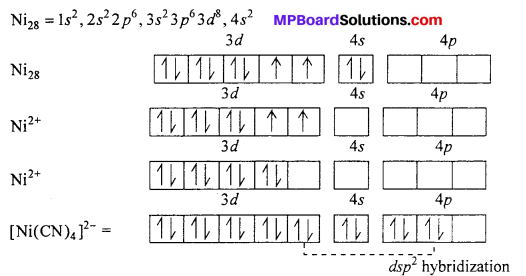Co-ordination number of Ni2+ in this complex ion is 4 and experimentally it is found to be diamagnetic. This is possible if it does not have unpaired electron i.e., $$3 d_{z^{2}}$$ gives its electron to 3dx2-y2 Now $$3 d_{z^{2}}$$, 4s, 4px and 4py orbital hybridizes forming four dsp2 hybrid orbital oriented along the comer of a planar square.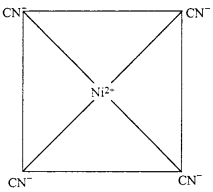These hybrids accept lone pair of electron from four CN ion forming σ – bonds. Now there is no unpaired electron in it. Therefore, [Ni(CN)4]2- is diamagnetic.

Question 11.
What is effective atomic number (EAN) ?
In co-ordination compounds, sum of electrons present in central metal ion and electrons received in bond formation is called effective atomic number.
EAN = Atomic number – Electrons lost in ion formation + Electrons obtained in bond formation
In K4[Fe(CN)6]EAN for Fe = 26 – 2 + 12 = 36.

Question 12.
Write the I.U.P.A.C. names of the following compounds :
(i) (a) [Hgl4]2-
(b) [Ag (CN)2]
(c) [Fe (C5H5)2l
(d) K [Ag (CN)2
(ii) What is Zeise’s Salt and Ferrocene ? Explain with structure.
(i) (a) Tetraiodomercurate (II) ion.
(b) Dicyanoargentate (I) ion.
(d) Potassium dicyano argentate (I).
(ii) (a) Zeise’s salt K [PtCl3 – n2 (C2H4):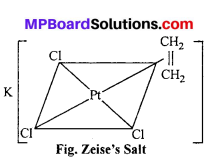This salt was prepared by Danish pharmacist Zeise in 1830. it is one of the compound of transition metals which was prepared earlier. The plane of ethylene molecule and the C = C axis are perpendicular to the expected bond direction of the central atom.

(b) Ferrocene Fe (n5 – C5H5)2: It is an orange-yellow coloured compound. Kealy and Pauson reported it in 1951. It has sandwich structure in which iron atom is in between two cyclopentadienyl rings. The planes of the rings are parallel so that all the carbon atoms are equidistant from iron atom.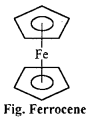Question 13.
What are chelates ? Give example. Write importance of Chelate.
Chelates is a multidentate ligand which co-ordinates with central metal atom to form ring compound, the process is called chelation.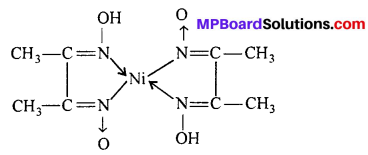Importance of chelate ligands :

1. In qualitative analysis, for the detection of some elements.
2. They are used in the separation of lanthanides and actinides.
3. In softening of hard water.

Question 14.
What is the difference between primary and secondary valency ? Give example also.
Primary valency is ionisable while secondary valency does not ionise. Primary valency is represented in figure by solid lines and secondary valency by broken or dotted lines.
Example : [Co (NH3)6] Cl3, primary valency is 3 and secondary valency is 6.

Question 15.
What is the importance of coordination compound in extraction of metals ?
In metallurgy, extraction of metals like gold and silver is down by forming complexes. Metals are converted into soluble cyanide complexes by treating with dilute cyanide solution. These soluble complexes liberates metals when treated with electropositive metals like zinc.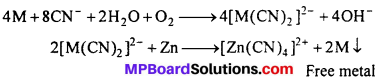Question 16.
Write I.U.P.A.C. name of the following :
(a) [CU(NH3)4]SO4
(b) [Cr(H2O)6 ]Cl3
(c) [Pt(NH3)6]Cl4
(d) K2[HgI4].
(a) Tetraammine copper (II) sulphate
(b) Hexaaquo chromium (III) chloride
(c) Hexaammine platinum (IV) chloride
(d) Potassium tetraiodomercurate (II).

Question 17.
Explain difference between Double salt and Complex compound.
Differences between Double salt and Complex compound :

Double salt

1. Addition compound in solid state but ionize in components when dissolved in the water.
2. These dissociate into constituent ions in aqueous solution.
3. The physical and chemical properties are similar to properties of constituents.
4. The nature of bond is ionic or electro-valent.

Complex compound

1. Stable in solid or in solution may be partialy ionize in to complex ion.
2. When dissolved in water form a complex ion.
3. Physical and chemical properties of complex compourids are quite different from constituents.
4. In complex compound, the bond present between the central metal ion and ligand is always co-ordinate.

Question 18.
Write the chemical formulae of the following :
(a) Trinitritotriamminecobalt (III)
(b) Tris (ethylenediamine) Chromium (III) chloride.
(c) Pentacarbonyliron (0)
(d) Tetrachloroplatinate (II) ion.
(a) [Co(NH3)3(ONO)3]
(b) [Cr (en)3]Cl3
(c) [Fe (CO)5]
(d) [Pt Cl4]2-

Question 19.
Write IUPAC name of the following :

1. K4[Ni(CN)4]
2. H2[CUCl4]
3. [Ag(NH3)2]Cl
4. [Ni(CO)4].

1. Potassium tetracyanonickelate (0)
2. Hydrogen tetrachloro cuprate (II)
3. Diammine silver (I) chloride.
4. Tetra carbonyl nickel (0).

Question 20.
Explain Linkage and Ionisation isomerism with example.
Linkage isomerism : Linkage isomerism occurs when different atoms of the ligand are attached to the central metal ion. The structure obtained are called linkage isomers. Such type of ligands are called ambidentate ligand.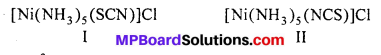In structure I Ni2+ is linked by thiocyanate sulphur and in structure II it is linked by nitrogen atom.
Ionisation Isomerism : This type of isomerism is shown by such compounds which have same composition but liberate different ions in solution.
[Co (NH3)5 Br]SO4 – It liberates SO2-4 ions.
[Co(NH3)5SO4]Br – It liberates Br ions.

Question 21.
(i) Write I.U.P.A.C. name of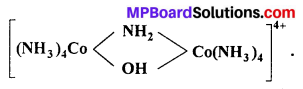(ii) Write the ligands and co-ordination number of the complex [Cr (NH3)4(ONO) Cl]NO3.
(iii) Write chemical formula of carbonate pentaammine cobalt (III) chloride.
(i) µ -amido- µ -hydroxobis-(tetraammine cobalt) (III) ion.
(ii) Ligands (a) NH3, (b) ONO, (c) Cl. Thus, number of ligands are 3 and co-ordination number is 6.
(iii) [CO(NH3)5CO3]Cl.

Question 22.
Write IUPAC names of the following complex compounds.

1. NH4[Cr(NH3)2 (NCS)4]
2. K2[Pt Cl6]
3. [CoCI(en)2NH3]++,
4. K[Pt(NH3)Cl5]
5. [Fe(CO)5].

1. Ammonium tetraisothiocyanatodiamminechromate (III)
2. Potassium hexachloroplatinate (IV)
3. Chlorobis-(ethylenediamine) amminecobalt (III).
4. Potassium pentachioroammineplatinate (IV).
5. Pentacarbonyl iron.

Question 23.
Write IUPAC name of the following :

1. Li(AlH4)
2. [Cr(NH3)6](NO3)3
3. (Cr(H2O)6]Cl3
4. K4[Fe(CN)6]

1. Lithium tetrahydridoaluminate (III)
2. Hexaammine chromium (III) nitrate
3. Hexaaquoch’romium (III) chloride
4. Potassium hexacyanoferrate (II).

Question 24.
Explain optical isomerism in co-ordination compounds.
Optical isomerism : This type of isomerism is observed in such similar compounds which are the mirror images of each other and are non-superimposable. They rotate the path of plane polarized light to the left (l) or to the right (d).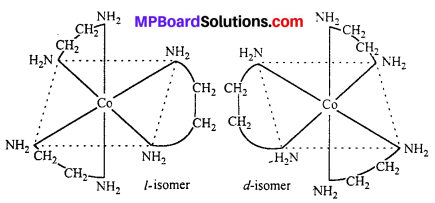Question 25.
Describe important applications of organometallic compounds.
Important applications of organometallic compounds are :

1. In medicine : Antiseptics like mercurochrome, mercury hydrix tincture are organometallic compounds of mercury.
2. In agriculture: Organometallic compounds of mercury like ethyl mercury chloride is used for treatment of seeds.
3. Antiknock compound : Tetraethyl lead (TEL) is an important antiknock compound.
4. In industries : Organolithium and organoaluminium compounds are used as catalyst for the manufacture of dyes and chemicals.
5. In chemical synthesis: Grignards reagent and organolithium compounds are useful in synthesis of organic compound.
6. Catalyst: Organometallic compounds of transition metals acts as heterogeneous catalyst, e.g., Wilkinson’s catalyst -(Ph3P)3 RhCl is used in hydrogenation of double bonds.Question 26.
Write IUPAC names of the following :

1. [Cr(H2O)6]Cl3
2. [Ag (NH3)2]CI
3. H2[CuCl4]
4. [CO(NH3)6]Cl3
5. K2[PtCl6]
6. [Pt Cl4(NH3)2]

1. Hexaaquachromium (III) chloride.
2. Diammine silver (I) chloride.
3. Hydrogen tetrachlorocuprate (II).
4. Hexammine cobalt (III) chloride.
5. Potassium hexa cloroplatinum (IV).
6. Diammine tetrachloroplatinum (IV).

Question 27.
Write IUPAC name of the following co-ordination compounds :

1. K[Ag(CN)2]
2. K4[Fe(CN)6]
3. [Ag(NH3)2]Cl
4. [Cr(NH3)6]Cl3.

1. Potassium dicyanoargentate (I).
2. Potassium hexacyanoferrate (II).
3. Diamminesilver (I) chloride.
4. Hexamminechromium (III) chloride.

Question 28.
Explain ionization isomerism and hydrate isomerism in coordination complexes giving suitable example.
Ionization isomerism : This type of isomerism is shown by such compounds which have same composition but liberate different ions in solution.
Example : [Co(NH3)5Br]SO4 (dark violet). It liberates SO2-4 ions in solution, whereas [Co(NH3)5 SO4] (red) liberates Br ions in solution. Both of these are ionization isomers.
Hydrate isomerism : This type of isomerism is shown by such compounds which differ in the number of molecules of water inside the coordination sphere.

Example : The three hydrate isomers of are as follows :

1. [Cr(H2O)6]Cl3 (violet)
2. [Cr(H2O)5Cl]Cl2.H2O (green)
3. [Cr(H2O)4Cl2]Cl.2H2O (darkgreen).

Question 29.
Write chemical formulae of the following co-ordination compounds :
(a) Hexammine cobalt (III) chloride
(b) Tetrammine copper (II) sulphate
(c) Tetrammine platinum (II) chloride
(d) Potassiumhexacyanoferrate (II).
(a) [CO(NH3)6]Cl3
(b) [Cu(NH3)4]SO4
(c) [Pt(NH3)4]Cl2
(d) K4[FeII(CN)6].

Question 30.
Write chemical formulae of the following co-ordination compounds :
(a) Chloropentammine cobalt (II) chloride
(b) Potassiumdicyanoargentate (I)
(c) Hexaquachromium (III) chloride
(d) Tetracyano nickelate (I)
(a) [CO(NH3)5Cl]Cl2
(b) K[Ag(CN)2]
(c) [Cr(H2O)6]Cl3
(d) [Ni(CN)4]2-.Question 31.
Write chemical formulae of co-ordination compounds :
(a) Hexammineplatinum (IV) chloride
(b) Potassiumhexacynoferrate (III)
(c) Dichlorodiammine platinum (II)
(d) Sodium pentacyanonitrosyl ferrate (III)
(a) [Pt(NH3)6]Cl4
(b) K3[Fe(CN)6]
(c) [Pt(NH3)2Cl2]
(d) Na2[Fe(CN)5NO]

Question 32.
Write IUPAC name of the following :
(a) [Pt(NH3)2Cl2]
(b) K3[Fe(CN)6]
(c) [CO(NH3)6]Cl3
(d) Pt[(NH3)6]Cl4
(e) CuCl2-4.
(a) Diachlorodiammine platinum (II)
(b) Potassium hexacyanoferrate (III)
(c) Hexammine cobalt (III) chloride
(d) Hexammine platinum (IV) chloride
(e) Tetrachlorocuprate (II).

Question 33.
Write IUPAC name of the following :
(a) K4[Fe(CN)6]
(b) [Cr(NH3)6(NO2)3]
(c) [Ni(CN)3]CI3
(d) K2[Pt(Cl)6]
(e) Ni (CO)4 U.
(a) Potassium hexacyanoferrate (II)
(b) Trinitrohexammine chromium (III)
(c) Tricyanonickel (III) chloride
(d) Potassiumhexachloro platinate (IV)
(e) Tetracarbonylnickel (0).

Question 34.
[NiCI4]-2 is paramagnetic while [Ni(CO)4] is diamagnetic though both are tetrahedral. Why ?
In [NiCl4]-2, Ni is +2 oxidation state with the configuration 4s03d8. Cl is weak ligand. It cannot pair up electrons in 3d- orbitals. Hence, it is paramagnetic. In [Ni(CO)4], Ni is in zero oxidation state with the configuration 4s2 3d8. In the presence of CO ligand the 45 electron shift to 3d to pair up 3d electrons. Thus, there is no unpaired electron present. Hence, it is diamagnetic.

Question 35.
How will you differentiate [Co(NH3)5Br]SO4 between and [Co(NH3)5SO4]Br ?
Both these complexes are ionisation isomers. First will give white precipitate (BaSO4) with BaCl2, second will give no precipitate with BaCl2. Similarly, second complex [Co(NH3)5SO4]Br will give yellow precipitate with AgNO3 whereas first will not give any precipitate.Coordination Compounds Long Answer Type Questions

Question 1.
Find the oxidation no. of central metal in the following :
(a) [Pt(NH3)3Cl3]
(b) [Zn(H2O)3OH]+
(c) Na4[Ni(CN)4]
(d) K2[Zn(OH)4]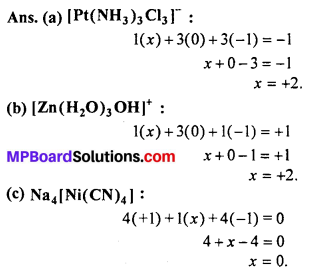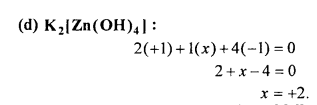Question 2.
Give methods of preparation of following (one method for each):
(i) Tetrabutyl tin
(iii) n-butyl lithium
(iv) Ferrocene
(v) Nickel tetracarbonyl
(vi) Zeise’s salt.
(i) Tetra butyl Tin: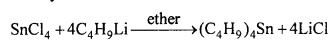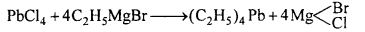(iii) n-butyl lithium: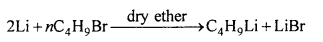(iv) Ferrocene: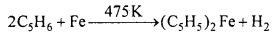(v) Nickel tetracarbonyl: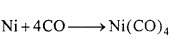(vi) Zeise’s salt: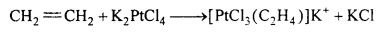Question 3.
Explain the structure of [Ni(CO)4] on the basis of valence bond theory.
Structure of [Ni(CO)4]: In nickel tetracarbonyl oxidation state of nickel atom is zero (0). Electronic configuration of Ni is 4s2 3d8 or 3d10. As a result of sp3 hybridization four sp3 orbitals are formed in tetrahedral form which are vacant. Four CO molecule join with them, as a result of which tetrahedral nickel tetracarbonyl molecule is formed.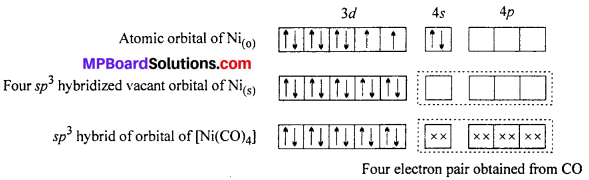Question 4.
Explain the structure of [Zn(NH3)4]2+ on the basis of valence bond theory.
Structure of [Zn(NH3)4]2+ Tetraammine zinc (II) ion : Outer electronic configuration of zinc (Z = 30) in ground state is 3d10 4s2. In this complex zinc is in + 2 oxidation state with the outer electronic configuration of 3d10.
The 3d orbital being completely filled does not take part in hybridization. The vacant 4s and 4p orbitals hybridize and form four hybridized sp3 rorbitais directed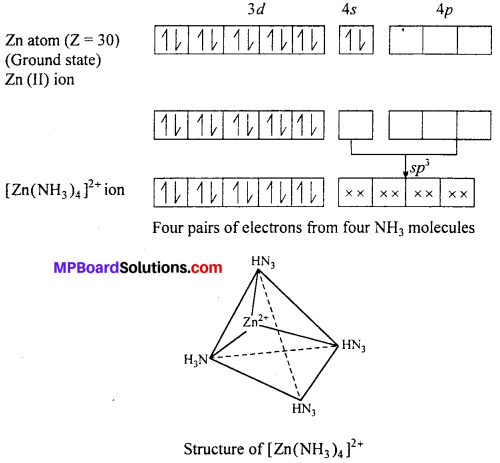towards the four comers of a tetrahedron. The four lone pairs from four NH3 overlap with these sp3 orbitals and forms four cr-bonds. The compound is diamagnetic as it does not contain unpaired electrons.

Question 5.
Explain optical isomerism with the example of tetrahedral and octahedral co-ordinate compounds.
Optical Isomerism : Optical isomers are sensitive to plane polarised light. When light passed through the solution of optically active substances these rotates the light-plane in clockwise or anticlockwise direction.

This type of isomerism is exhibited by the substances which are mirror image of each other but cannot be superimposed. Optical isomerism is shown by the substances which have minimum one assymmetric carbon atom.

The compound which rotate plane polarized light in left side are called l-isomers and which rotate in right side called d-isomers. These types of isomerism are generally found in tetrahedral complex [-CN = 4] and octahedral complex [-CN = 6].

(i) Tetrahedral complex : [As (CH3) (C2H5) (S) (C6H4COO)]2+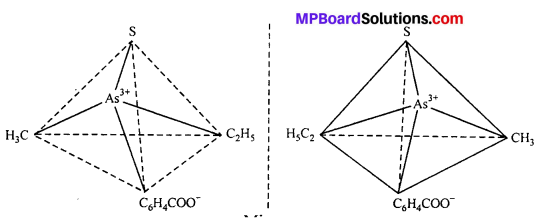(ii) Octahedral complex : [CO(en)2]3+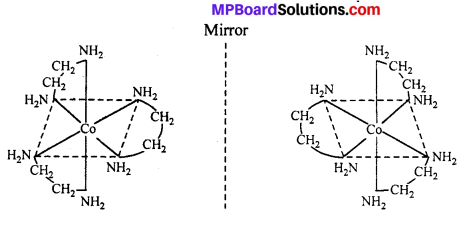Question 6.
Write the application of complex compounds in the extraction of metals and detection of metal ions.
(i) In extraction of metals: Silver and gold ores are treated with NaCN solution and form soluble complex.
(a) Silver glance (Ag2S) dissolves in NaCN solution and forms soluble complex Na- [Ag(CN)2] from which Ag is precipitated by adding Zn dust.
Ag2S + 4NaCN → 2Na[Ag(CN)2] + Na2S
2Na [Ag(CN)2 ] + Zn → Na2 [Zn (CN)4] + 2 Ag ↓

(b) Gold is found in native state. Its powdered ore is kept in NaCN or KCN solution for 12 to 24 hours. Au dissolves by forming soluble complex K[Au(CN)2], Now zinc sharings are added to precipitate Au.
4AU + 8KCN + 2H2O + O2 (from air) → 4K[Au(CN)2] + 4KOH
2K[AU(CN)2] + Zn → K2 [Zn(CN)4] + 2Au ↓

(ii) In the detection of metal ion : In the qualitative analysis of Ni2+ a scarlet pink colour complex is obtained with (D.M.G.) dimethyl glyoxine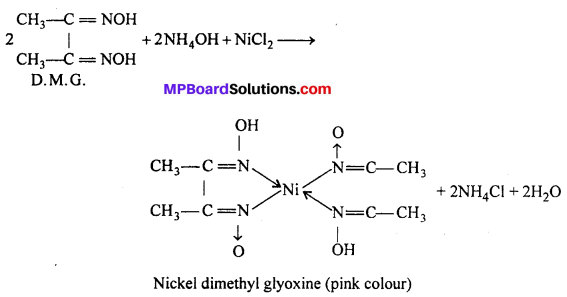Question 7.
Explain crystal field theory.